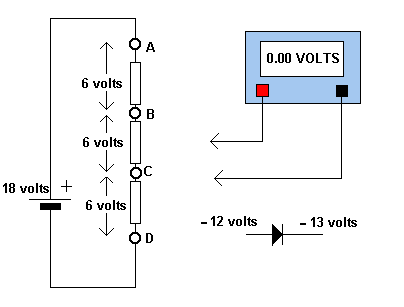Electronic Circuits and Tutorials
Tutorials
Dictionaries
Projects
Discover
Home > Electronics Tutorial > Test and Measurement > With Respect To - Tutorial
Test and Measurement in Electronics

# With Respect To - Tutorial

In most cases, when measuring DC voltages in an electronic circuit, the black negative lead is clipped to the negative connection of the battery or supply. This leaves one hand free. The red lead is then used to measure the various voltages in the circuit. These voltages are therefore measured WITH RESPECT TO negative.wrt = with respect to

In the diagram, point A is 18 volts positive wrt to D. (this is actually the battery voltage).
Therefore D is 18 volts negative wrt A.

A is +12 wrt C and +6 wrt B.

D is - 6 wrt C and -12 wrt B.

B is +6 wrt C and +12 wrt D.

C is - 6 wrt B and -12 wrt A.

Looking at the diode. The anode is less negative than the cathode. Or in other words, it is more positive. The anode is positive wrt cathode by 1 volt.

Note: To report broken links or to submit your projects, tutorials please email to Webmaster